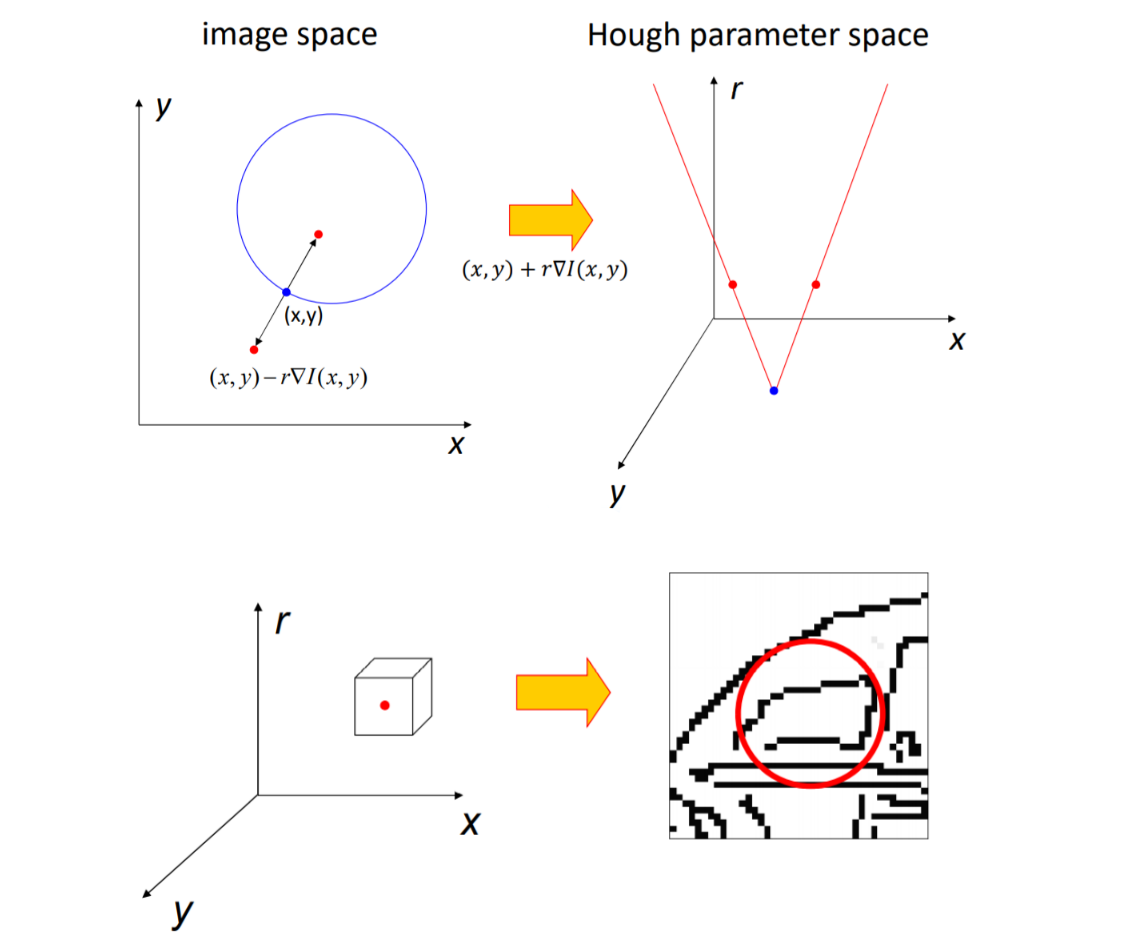# 拟合(Fitting)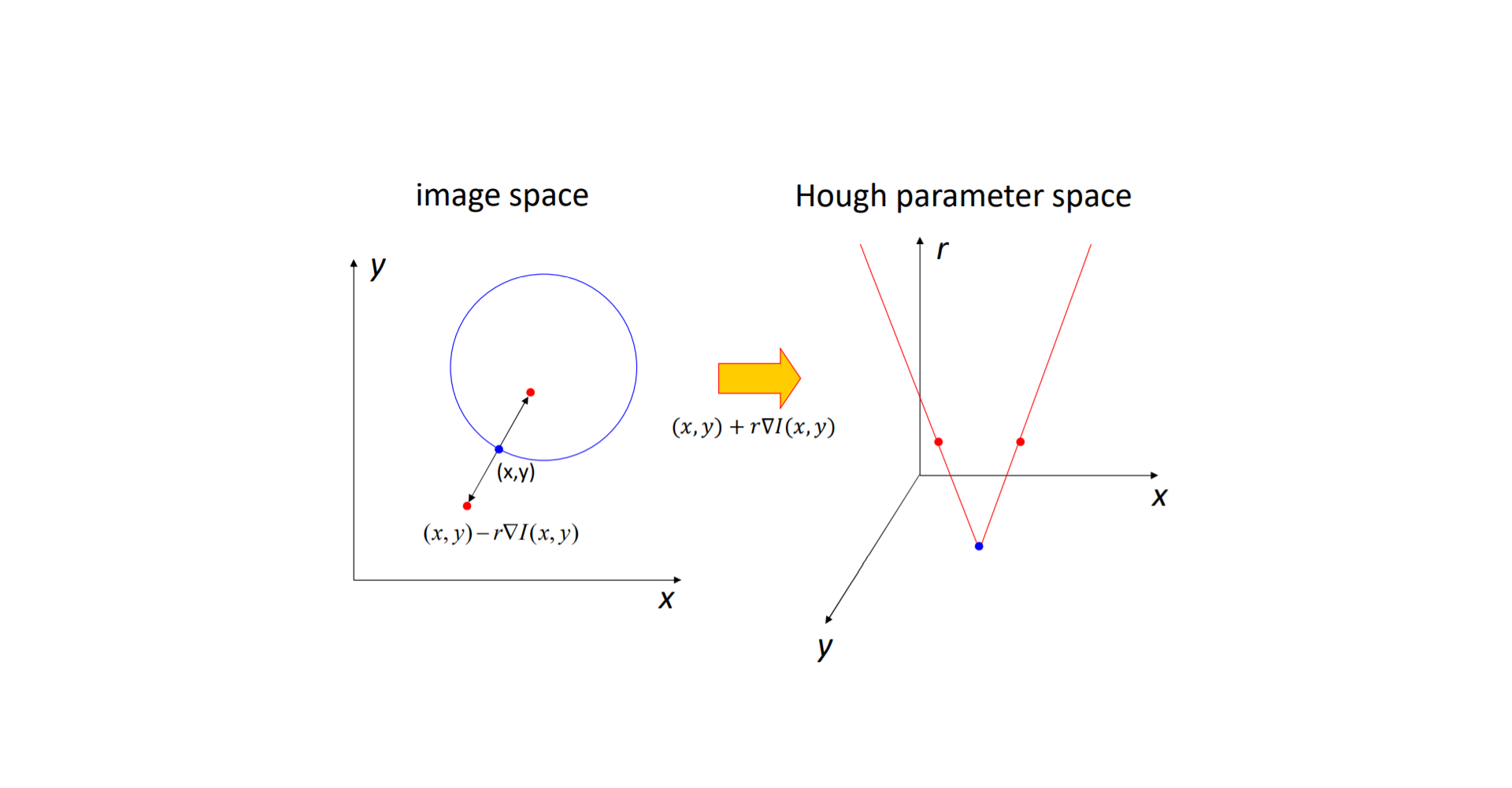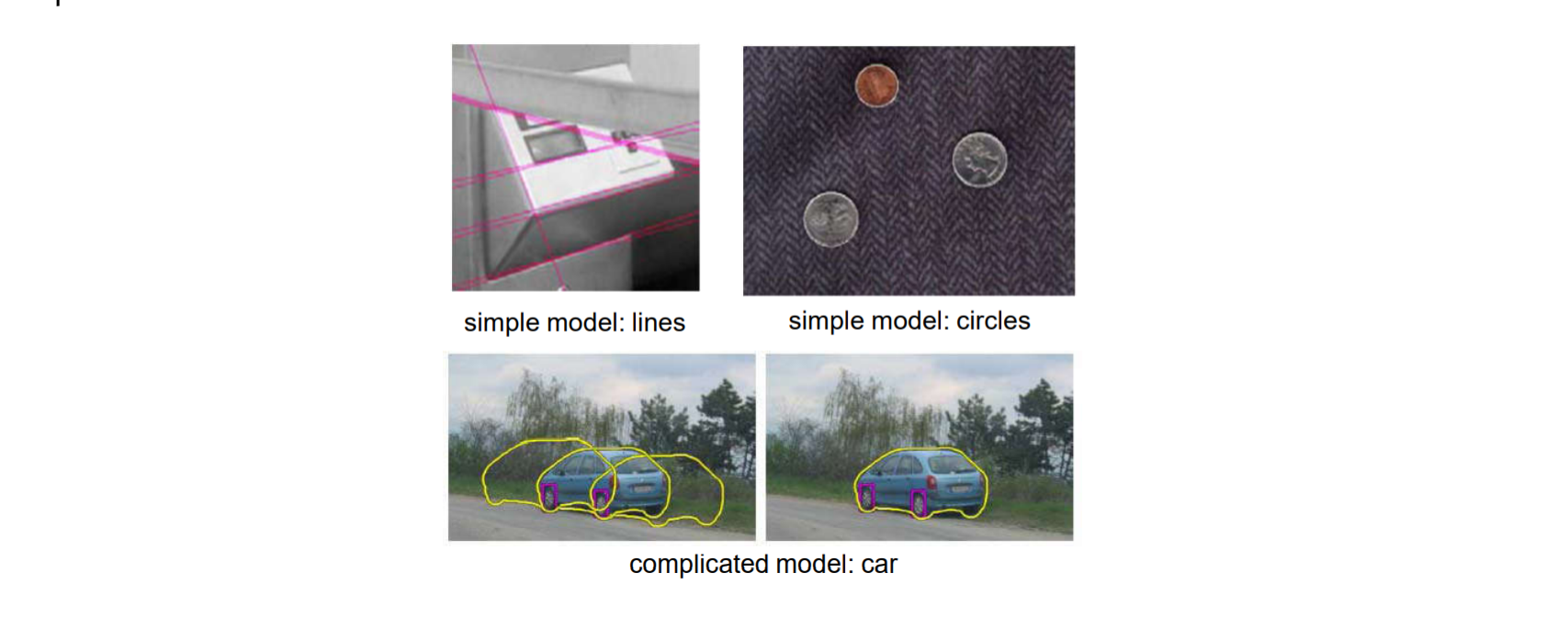## 难点1. 噪声：噪声的存在使拟合的模型偏离真实的线
2. 外点：在目标图形以外的线，如上图中的目标图形为“车”，左边的“栅栏”就是外点
3. 目标图形部分被遮挡，使部分图形消失

## 最小二乘（Least Square）

• 最小二乘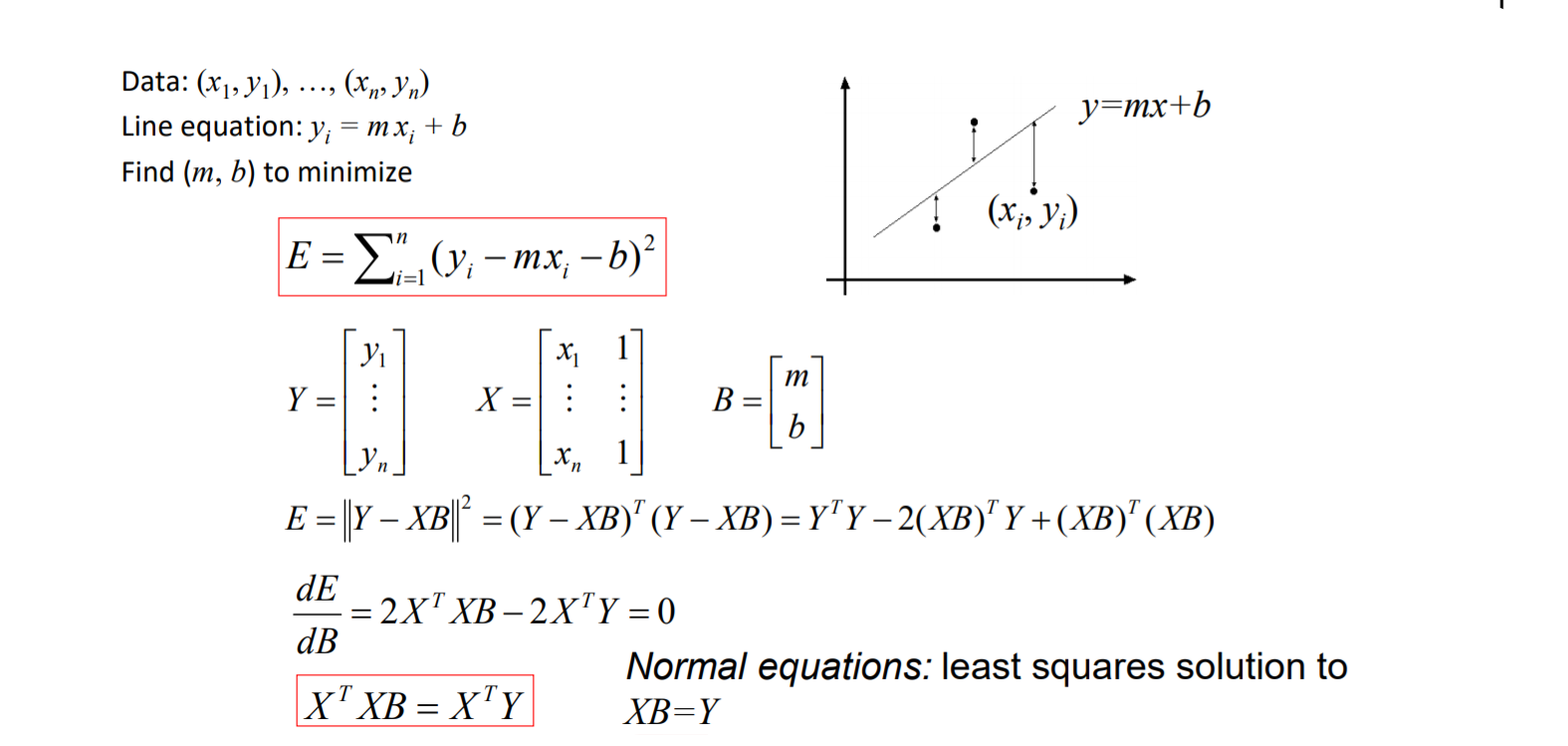• 权最小二乘 当拟合的直线是平行$y$轴时就无法按照上面的公式计算$E$（最小二乘对旋转没有效果）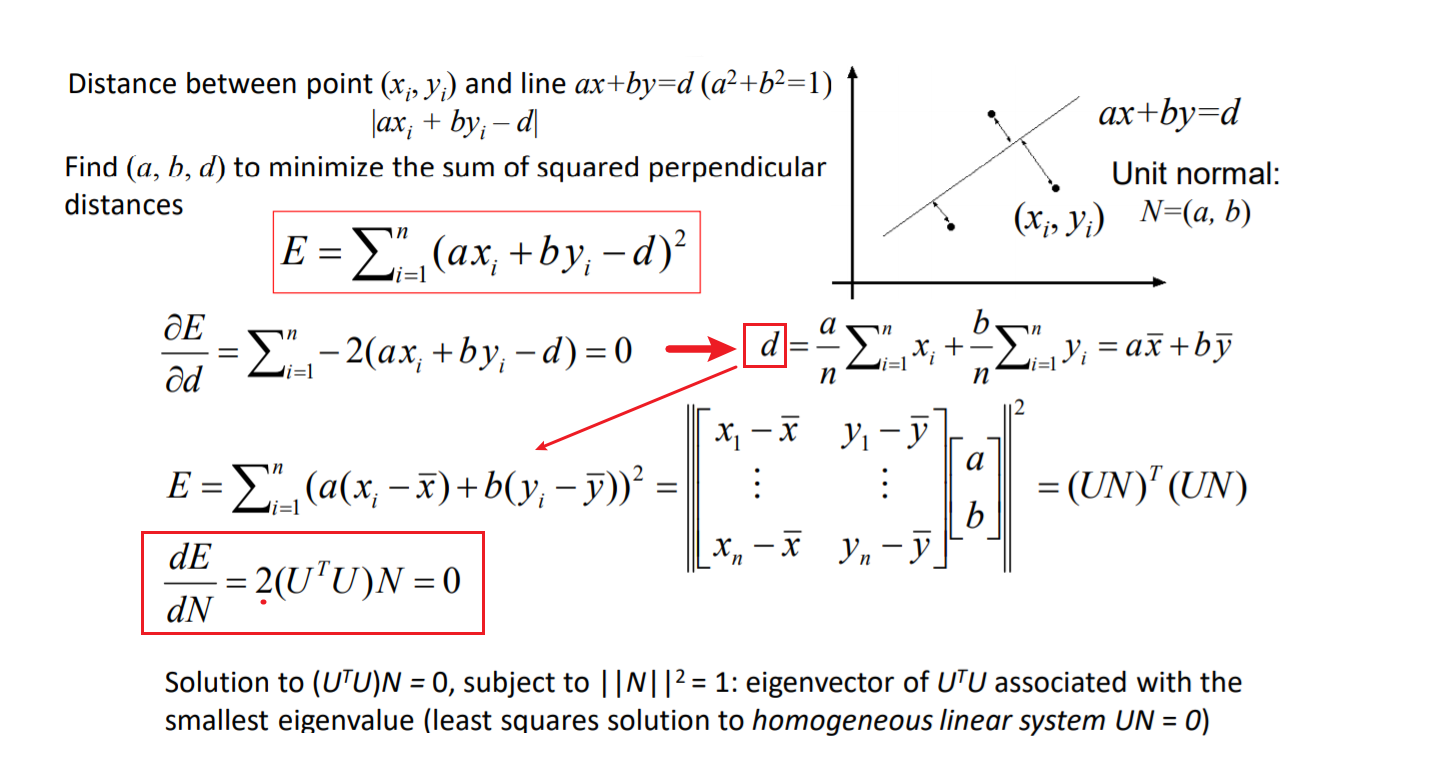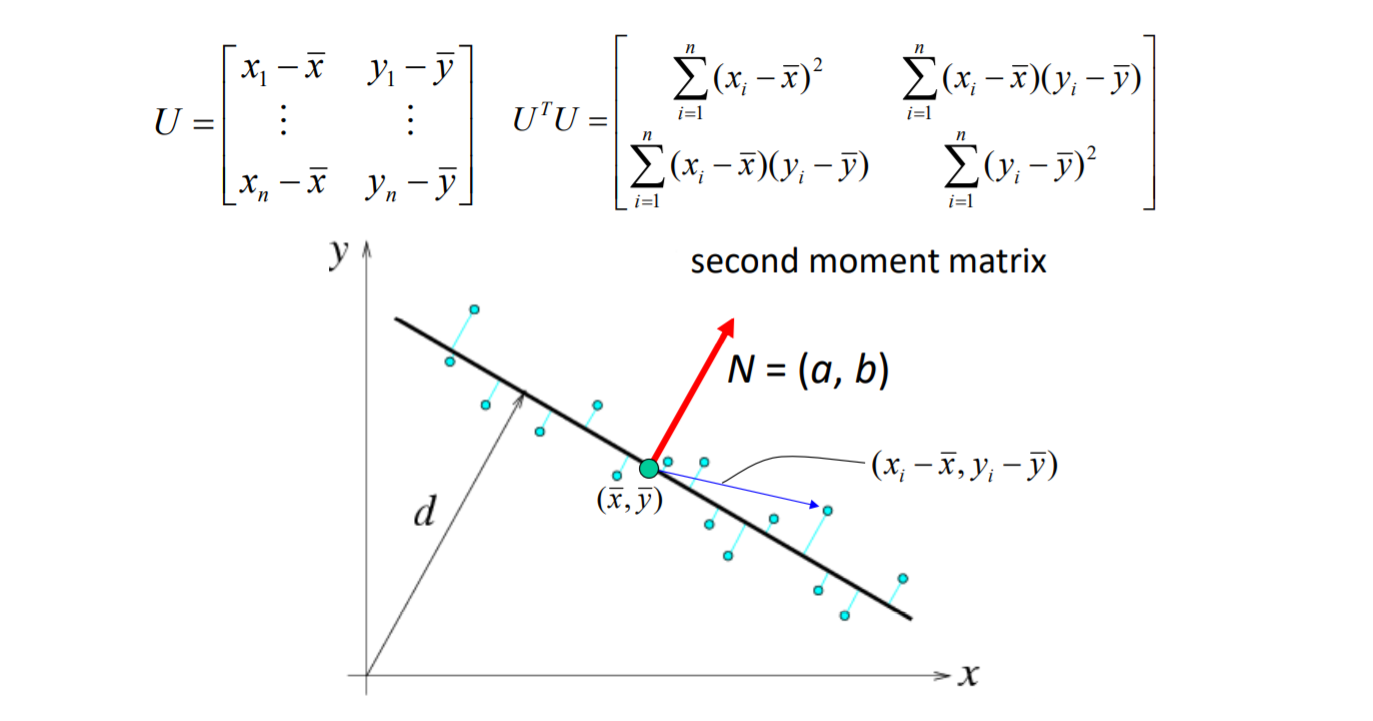• 极大似然估计

使用概率分布的思想来理解权最小二乘，概率越大拟合效果越好，极大似然估计，就是利用已知的样本结果信息，反推最具有可能（最大概率）导致这些样本结果出现的模型参数值，它提供了一种给定观察数据来评估模型参数的方法，即：“模型已定，参数未知”。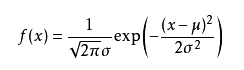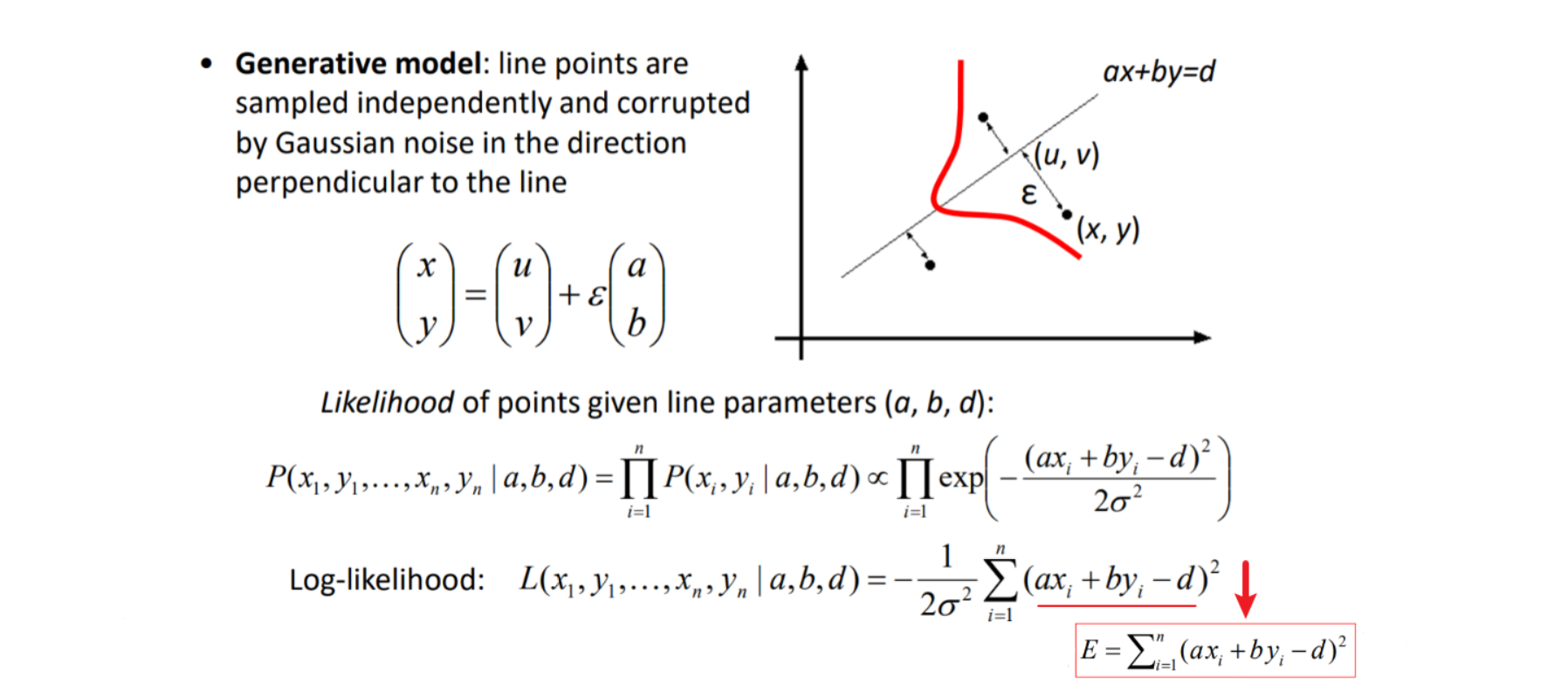## Roubst Fitting & RANSAC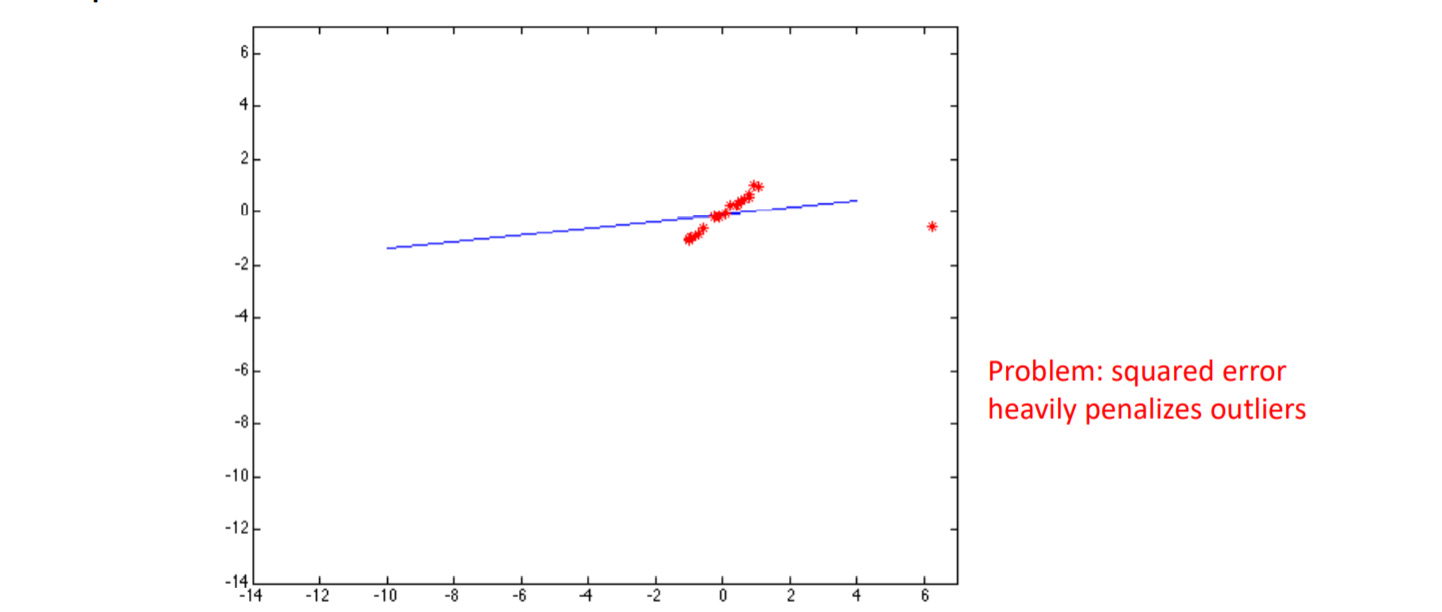### Roubst fitting（鲁棒拟合）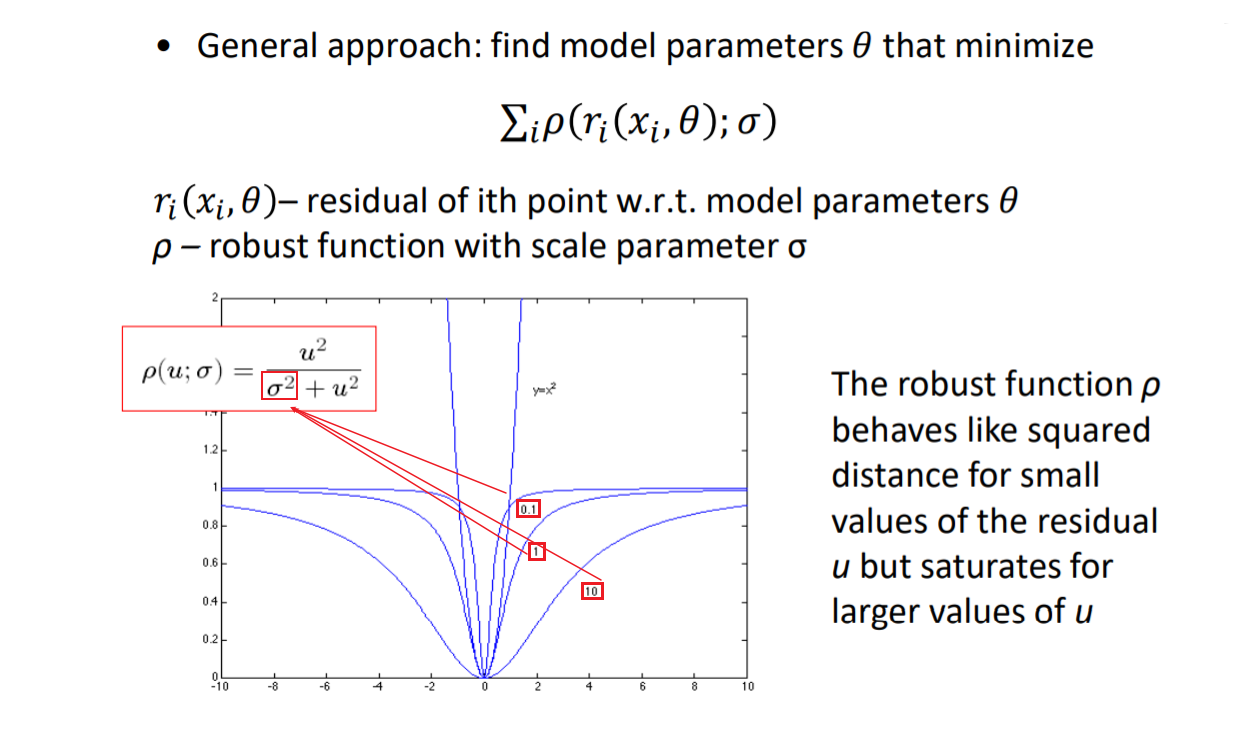• 鲁棒函数估计
1. 对于一个非线性的优化问题就不能使用前面的方程求解，需要使用迭代的方式求解类似于梯度下降
2. 先不考虑鲁棒拟合的问题，利用最小二乘得到一个初始解
3. 根据经验将尺度参数$\sigma$设置成$1.5$倍的平均残差
• 处理后的效果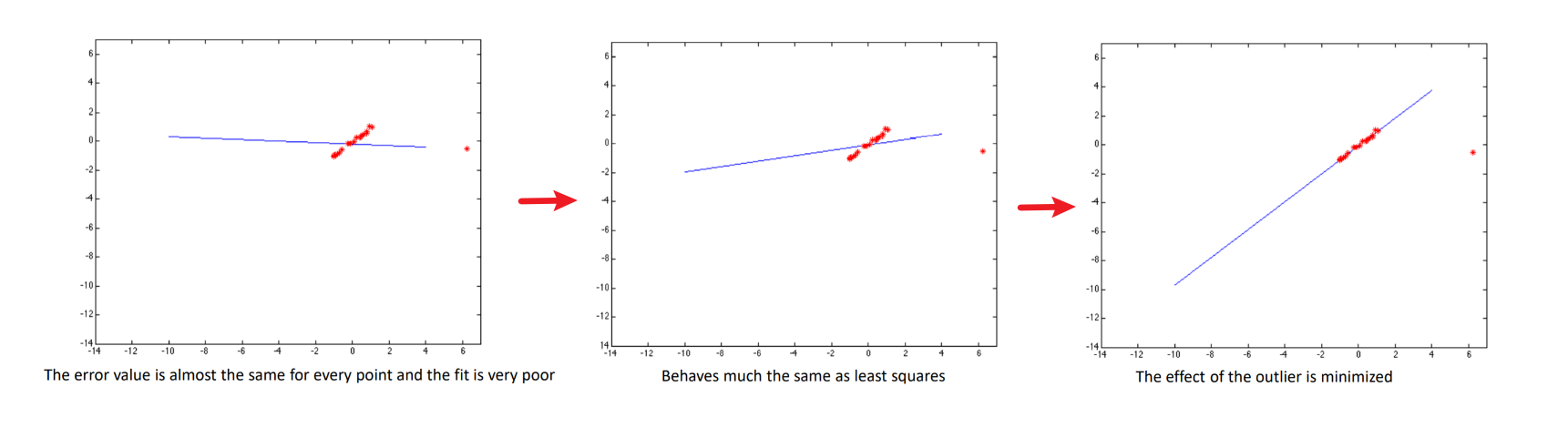### RANSAC（随机采样一致性）

• RANSAC
1. 选择一个最小的集合$s$（估计一条直线需要两个点）
2. 拟合出一个模型
3. 设置一个门限$t$
4. 用门限$t$内剩余的点给这个模型“投票”，即“离得近”就 得分
5. 重复上述过程，取“得分”最高的模型,设置迭代次数$N$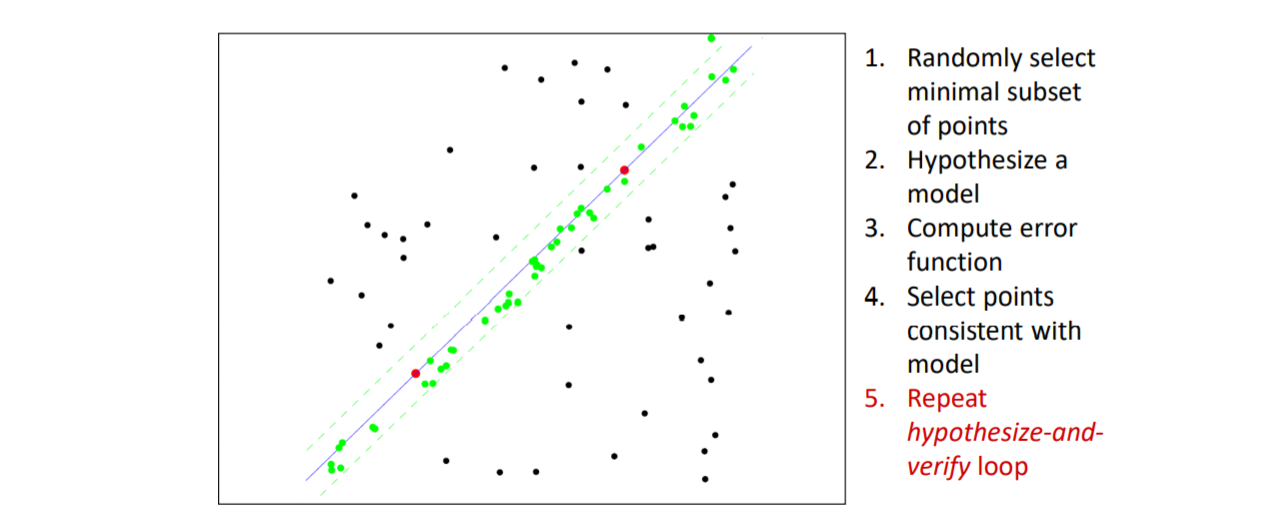• 选择参数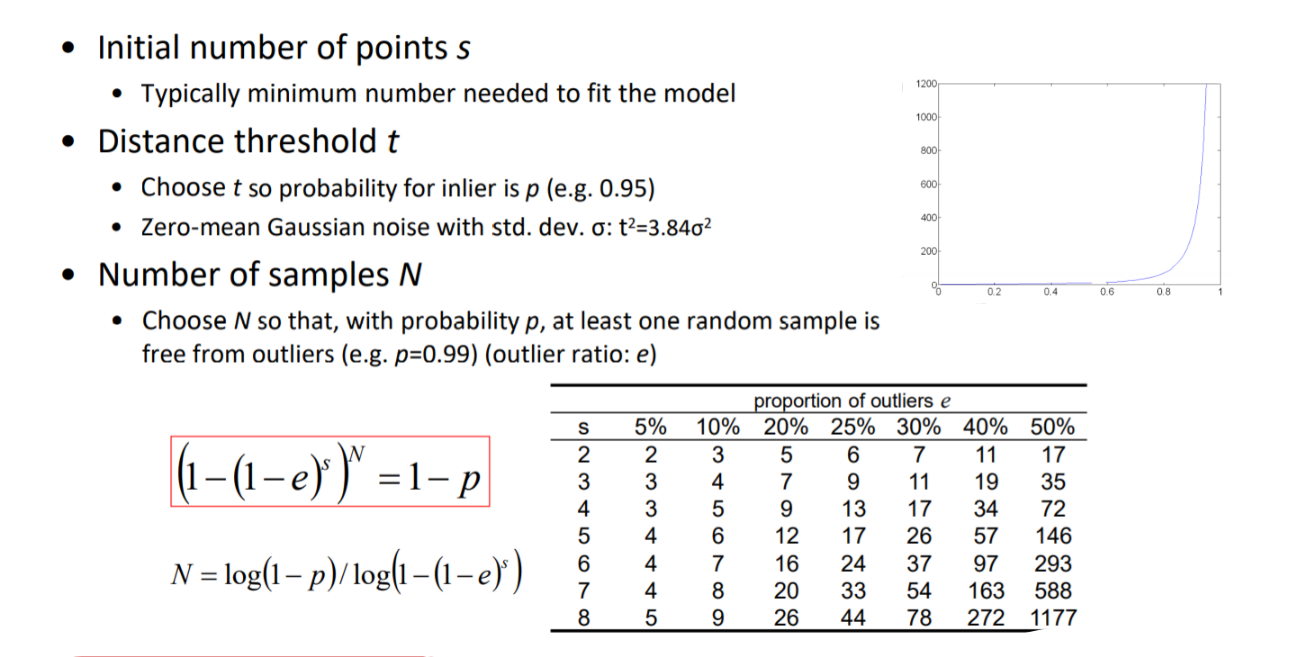$e$：外点率，$s$：模型的最小范围，$N$：最大迭代次数，$p$：正确率

• 自适应的参数提取 在实际问题中，只知道参数$t,s$,无法知道外点率$e$也就无法确认迭代次数$N$。 解决方法如下：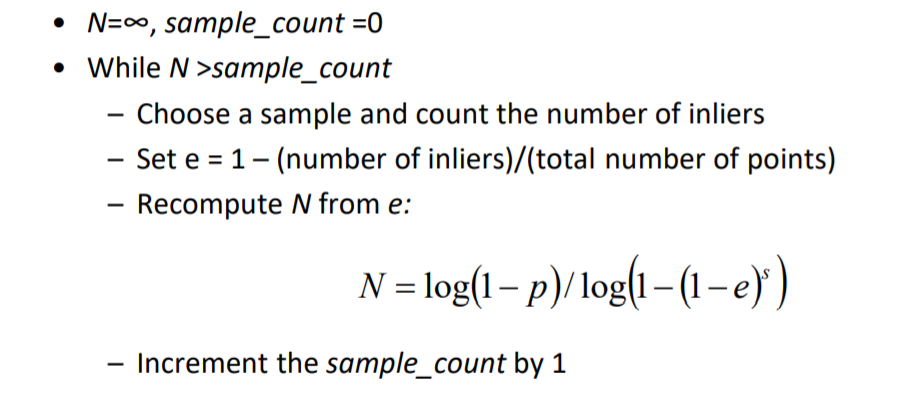• 使用RANSAC思想进行指纹识别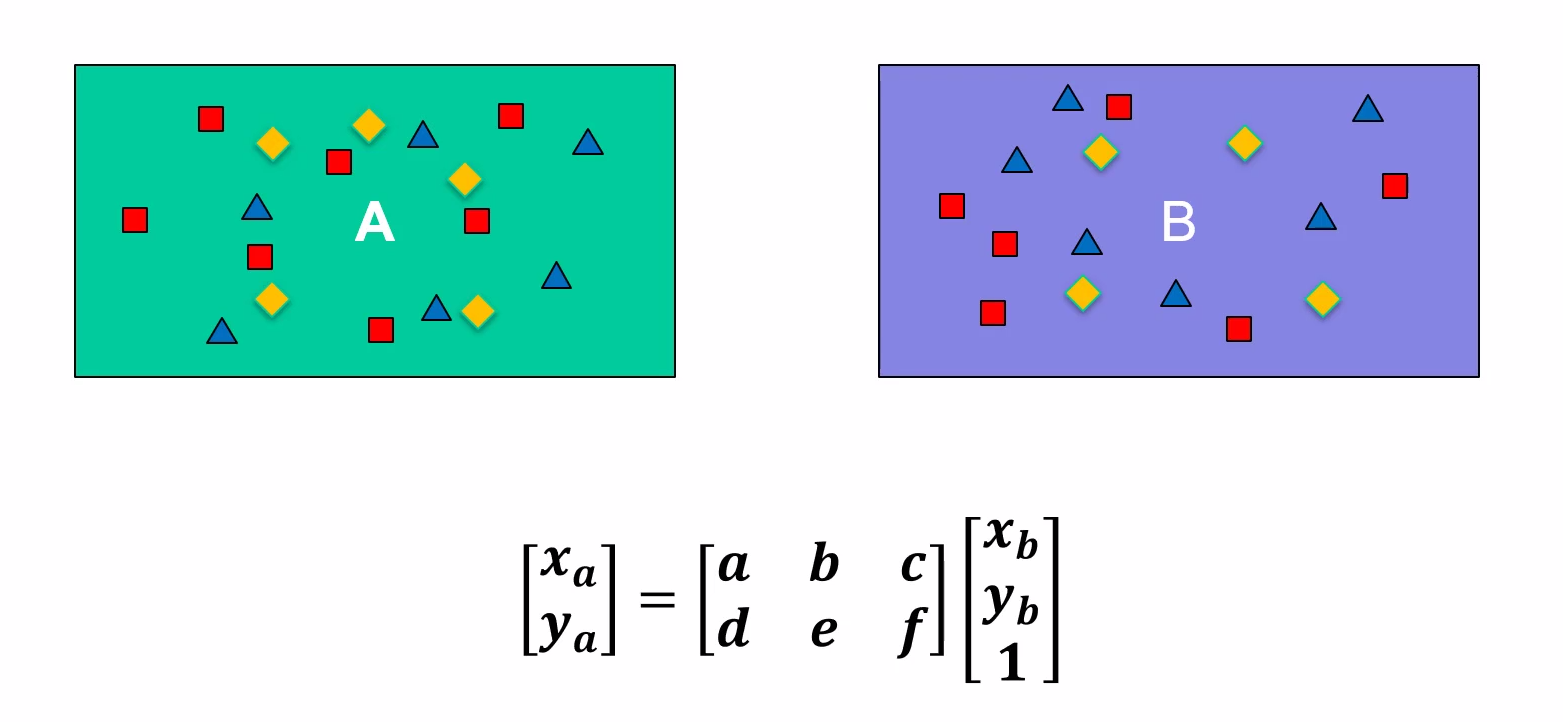## 霍夫变换（Hough Transfrom）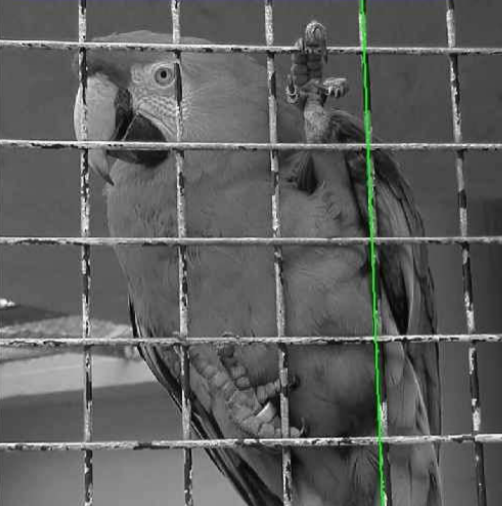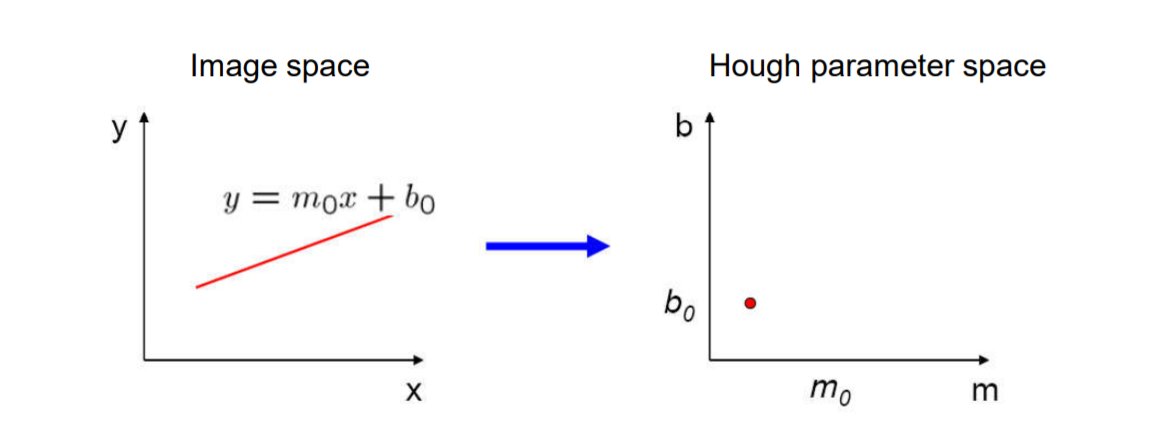• 一些例子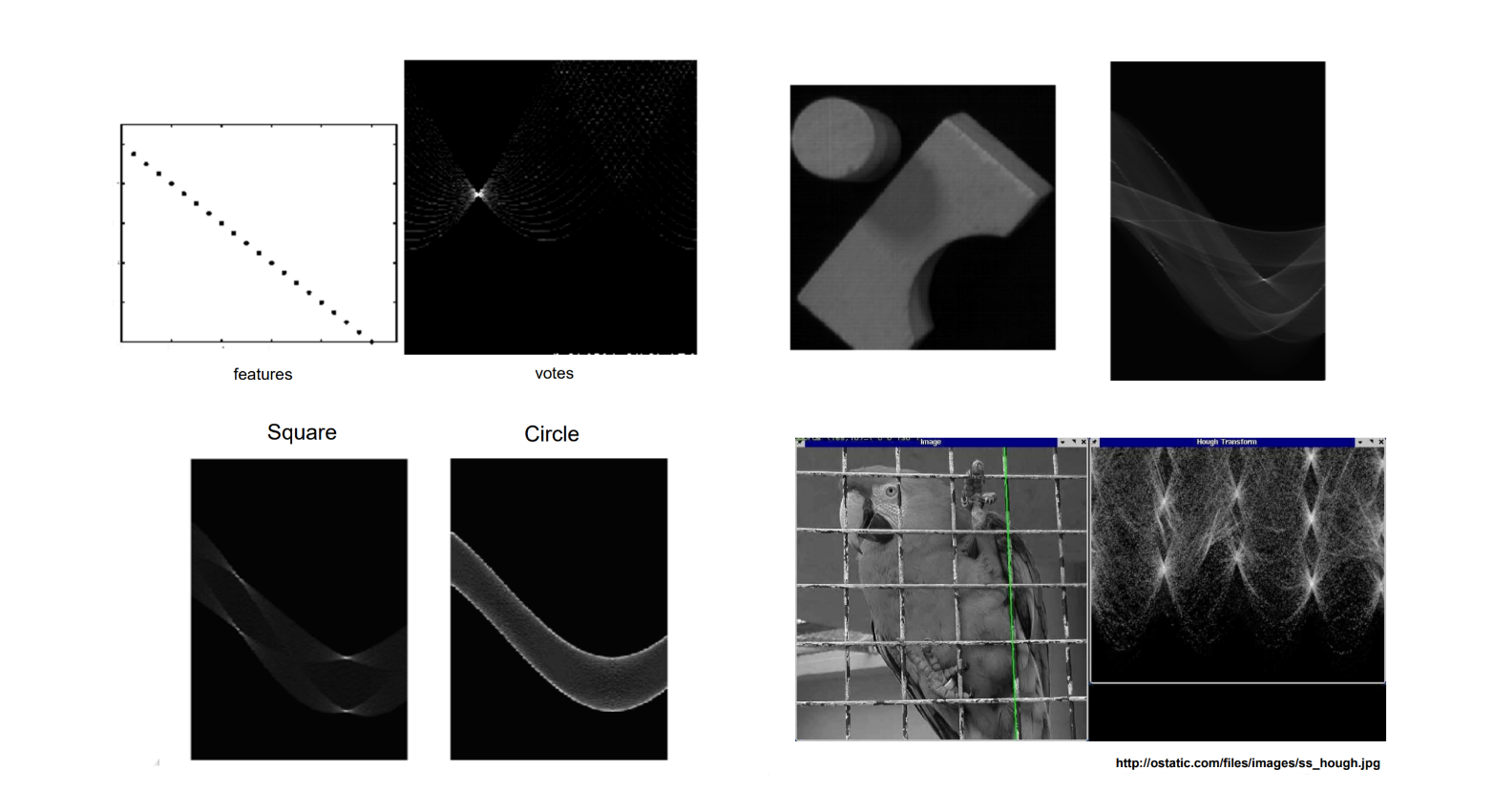• 霍夫变换处理噪声

在Candy算子中得到点时就知道了梯度方向，相应的边缘方向的范围就大概确认了，这是就是可以缩小$\theta$的范围，从而解决了噪声的影响，也简化了计算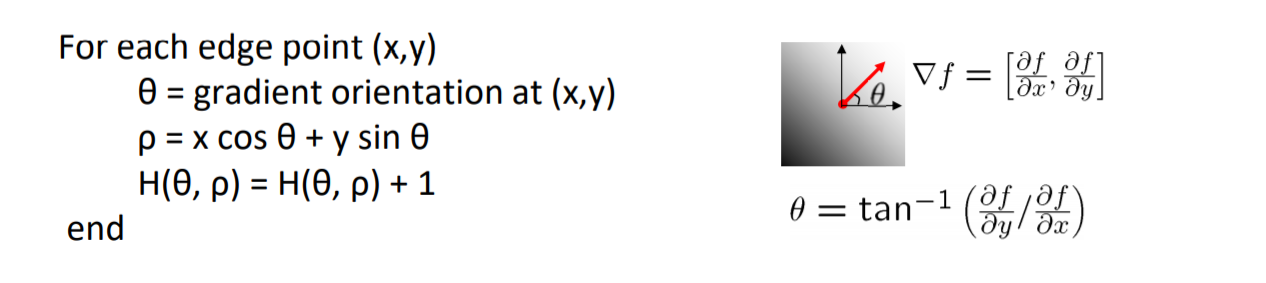• 霍夫变换拟合圆

确定一个圆需要圆心坐标$(x, y)$和半径$r$，有三个参数，参数空间就需要是一个三维空间，取圆上的一个点，则可以由梯度方向确定半径方向，穷举所用的$r$(大于0，小于图像长度)，遍历圆上的点对$r$进行投票，最后在参数空间会得到一个票数高的三维空间，这个三维空间的中的一点$(x,y,r)$就可以作为拟合的圆心和半径。